# DataWeave mapObject function: How to transform key/value pairs in an Object

We would like to thank MuleSoft Ambassador Joshua Erney for their contribution to this developer tutorial.

In this tutorial, you’ll learn some examples in which you can iterate through all the key/value pairs in an Object to change them to something else. `mapObject` is similar to `map` in the sense that this could be an equivalent to `for` or `forEach` if you come from a different development background. Since DataWeave is a functional programing language, the statements are not being executed in a sequence (like a `for` would).

To learn more about the differences between DataWeave as a functional programming language and imperative programming, check out the documentation.

You can try all of these examples with the DataWeave Playground. To learn more about it, check out this tutorial.

## Prerequisites

While not required to follow this tutorial, a good understanding of the basic DataWeave concepts would be preferred. You can check out these other tutorials if you feel a bit lost with some concepts:

## Syntax

We use `mapObject` when we want to change the keys and/or values on an Object to be something else. `mapObject` takes in an Object, and a lambda that takes in 3 parameters: a value (`V`), a key (`K`), and an index (`Number`); and returns a new Object. Finally, the entire function returns the transformed Object.

No matter what type is used to create Object keys, they are always coerced to type Key. Even if the lambda returned a Number, the keys of the output Object would ultimately be of type Key.

## Prefix Notation

This function is not commonly used with the prefix notation because of its complexity. But here you can find an example that transforms all the keys and values to upper case.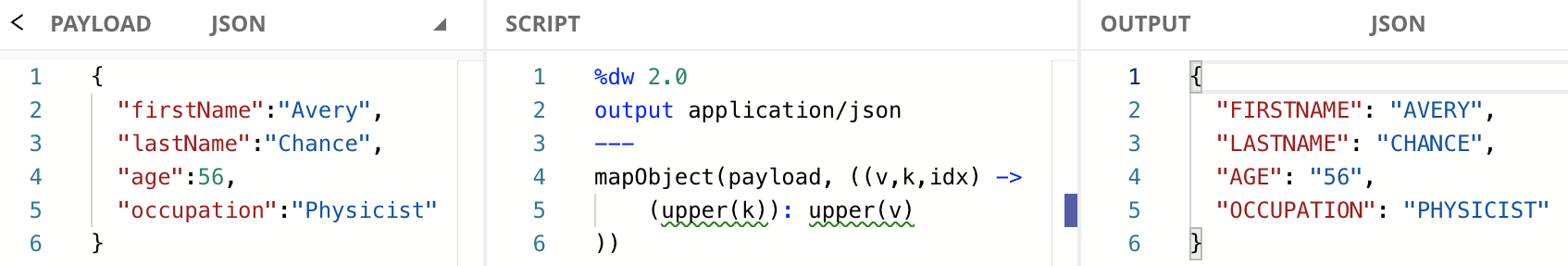The age was transformed from `56` to `"56"`. This is because the `upper` function returns a String, even if the input was a Number. To learn more about the `upper` function, check out the documentation.

## Infix Notation

This is the most used notation for this function because it makes it easier to read and understand.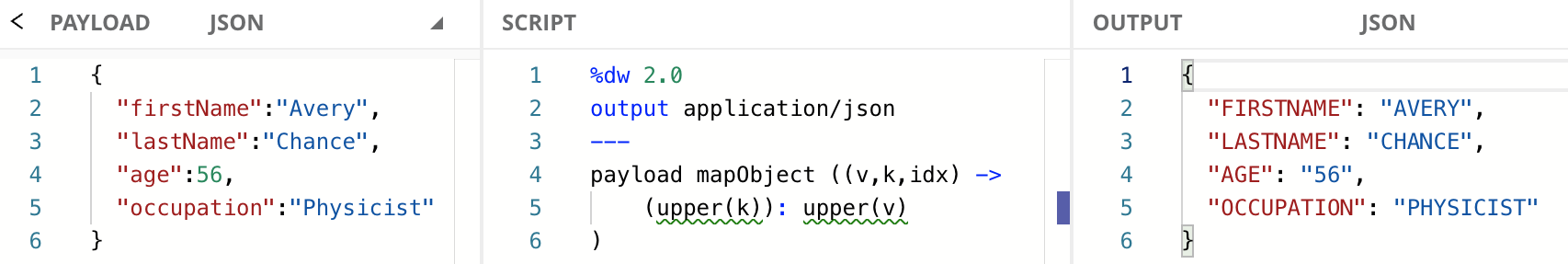When using the infix notation, you don’t have to include all the parameters in the lambda if you’re not using them. However, you do have to include the previous parameters (to the left) whether you’re using them or not. You can only remove the parameters to the right.

In this case, since `v` and `k` are the first and second parameters, we can also use the following code:

## Dollar-sign Syntax

You don’t always need to include the whole explicit lambda expression. You can use the dollar-sign syntax to reference the three arguments that are passed to the lambda (i.e., `v`, `k`, and `idx`). `\$` represents the first argument: the value from the key/value pair (`v`), `\$\$` represents the second argument: the key from the key/value pair (`k`), and `\$\$\$` represents the third argument: the index for each key/value pair (`idx`).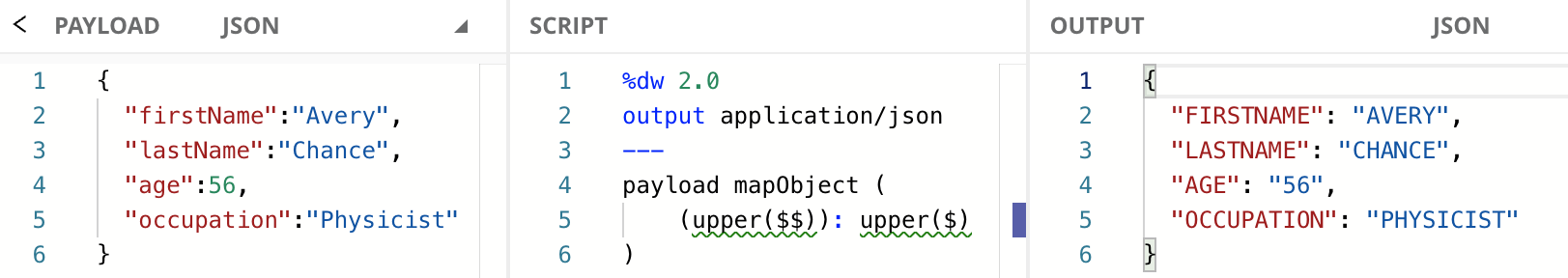## Transform Key/Value Pairs by Value

In the previous example, we ended up transforming the `age` field from a Number value (`56`) to a String value (`"56"`) by accident when we used the `upper` function. We can use `if/else` or `match/case` to only use the `upper` function if the given value is a String and leave the other values intact. In this example, we won’t modify the keys, just the values.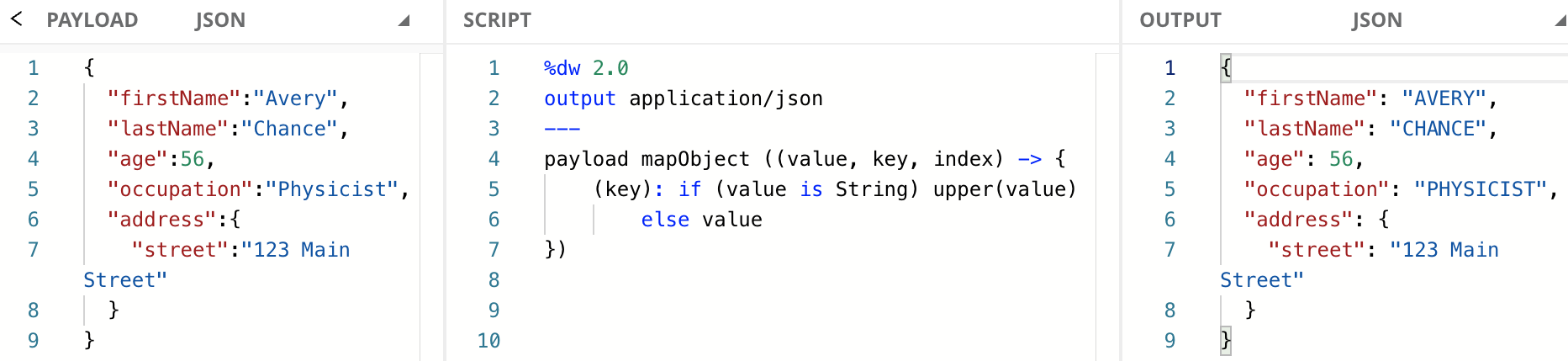Note how the `street` field’s value was not transformed into upper case. This is because the `mapObject` function only takes into account the first level of key/value pairs it finds. In this case, the `address` field’s value is an Object. The key/value pairs inside this Object won’t be transformed.

If you wanted to be able to read all the “leaf values” from the Object, check out the `mapLeafValues` function from the `Tree` module in the documentation.

When using the infix notation, you don’t have to include all the parameters in the lambda if you’re not using them. In this case, since we’re only using `key` and `value` we can also use the following code:

Finally, here is how the previous code would look like with the dollar-sign syntax:

You need to surround the `key` (or `\$\$`) parameter in parentheses to correctly reference the value of the key when using it as a Key. If you don’t do this, then the String `"key"` will be taken instead.

## Transform Key/Value Pairs by Key

Let’s say for example we wanted to change `"firstName"` and `"lastName"` to only be `"first"` and `"last"` accordingly. We could use the `replace` function to modify the keys containing `"Name"` and remove that String from the key.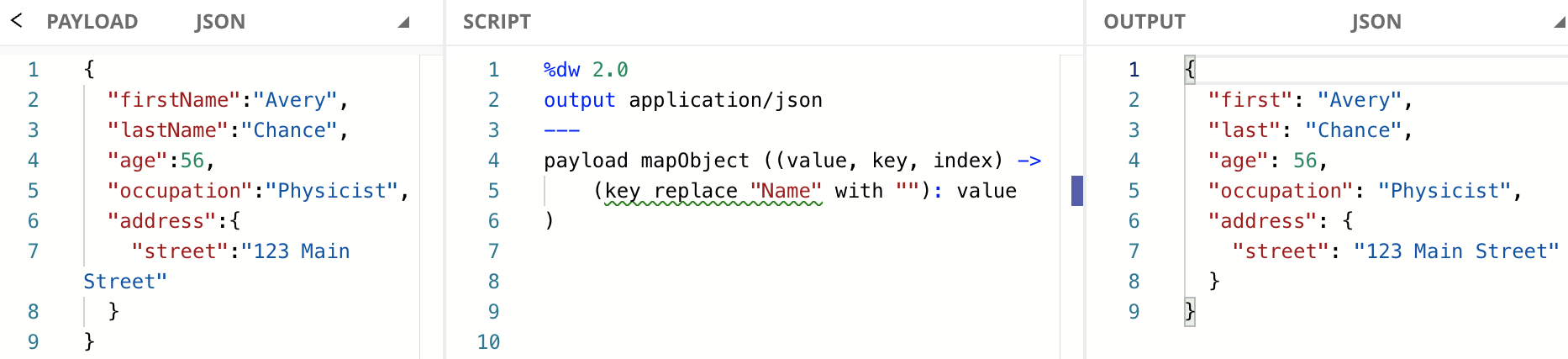If you’re not familiar with the `replace` function, you can use it to perform a String replacement. All the different ways to use `replace` are out of scope for this tutorial, but you can learn more about it in the documentation.

When using the infix notation, you don’t have to include all the parameters in the lambda if you’re not using them. In this case, since we’re only using `key` and `value` we can also use the following code:

Finally, here is how the previous code would look like with the dollar-sign syntax:

## Transform Key/Value Pairs by Index

Depending on your integration or your requirements, you may have to use this function to transform your payload into different output data. In this example, we are creating an Object with the index from each key/value pair that will contain another Object with each key and value.Notice how the parentheses around each key change the functionality of what is shown at the output. If we surround the parameter in parentheses, the value of the parameter is outputted (like `(index):` on line 5), while `key:` and `value:` (lines 6 and 7) are simply printed as hardcoded Strings.

Finally, here is how the previous code would look like with the dollar-sign syntax:

You need to surround the `index` (or `\$\$\$`) parameter in parentheses to correctly reference the value of the index when using it as a Key. If you don’t do this, then the String `"index"` will be taken instead.

## Next Steps

In this tutorial, you learned some examples in which you can iterate through all the key/value pairs in an Object to change them to something else. `mapObject` is similar to `map` in the sense that this could be an equivalent to `for` or `forEach` if you come from a different development background. Since DataWeave is a functional programing language, the statements are not being executed in a sequence (like a `for` would).

Continue your development journey with the rest of the tutorials to become a master in DataWeave.